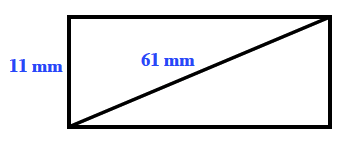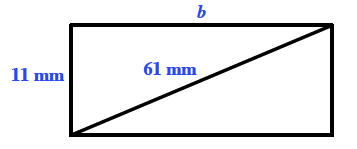### Home > CCG > Chapter Ch7 > Lesson 7.1.1 > Problem7-8

7-8.

A rectangle has one side of length $11$ mm and a diagonal of $61$ mm. Draw a diagram of this rectangle and find its width and area.

Use the Pythagorean Theorem to solve for $b$.
After solving for $b$, find the area of the rectangle.